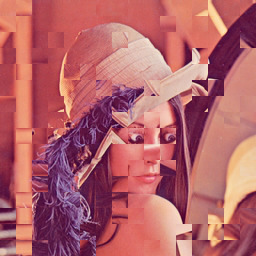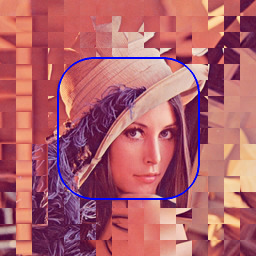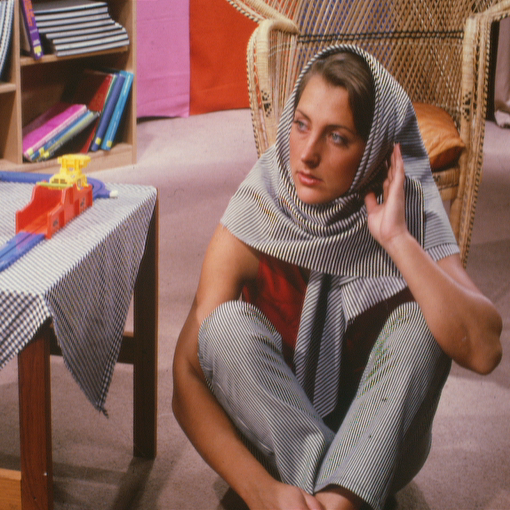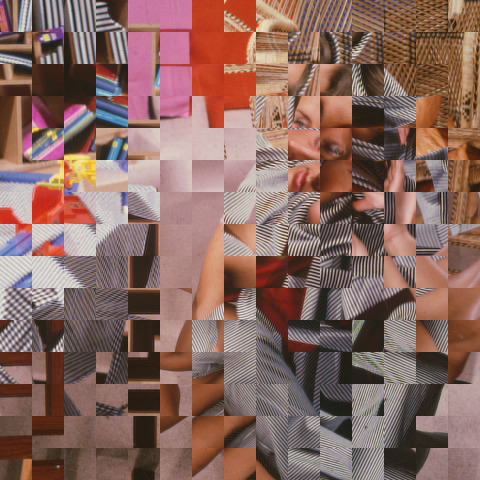## DICE

 Rotates each successive square-sized patch in the image.

### EXAMPLES

 Original:Arguments: (default)Original:Arguments: -p 35Original:Arguments: -c 128,128 -r 70,70 -R 35,35 -e blueOriginal:Arguments: -c 128,128 -r 70,70 -R 35,35 -e blue -nOriginal:Arguments: -c 128,128 -r 70,70 -R 35,35Original:Mask:Arguments: -e blueOriginal:Arguments: -s 32Arguments: -s 16What the script does is as follows: Crops the image to a multiple of the dimension Does a dimension-sized tiled crop of image Rotates the image randomly between 0, 90, 180 and 270 deg Montages all the tiles back to form the output image This is equivalent to the following IM commands wh=`convert -ping "\$infile" -format "%wx%h" info:` ww=`echo "\$wh" | cut -dx -f1` hh=`echo "\$wh" | cut -dx -f2` ww=`convert xc: -format "%[fx:\$dim*floor(\$ww/\$dim)]" info:` hh=`convert xc: -format "%[fx:\$dim*floor(\$hh/\$dim)]" info:` numw=\$((ww/dim)) numh=\$((hh/dim)) num=\$((numw*numh)) convert -quiet "\$infile" -crop \${ww}x\${hh}+0+0 +repage -crop \${dim}x\${dim} \$tmpA1 ( for ((i=0; i